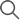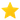# What Is The Probability Of Getting a Black Face Card in Poker?

## Table of content:

Poker involves a host of complex mathematical calculations based on probabilities. A good knowledge of numbers provides an essential basis for being a good poker player. For every decision that the player has to take, math and psychology will play a key role.

In this article, we will explain the importance of math in poker and give you a brief understanding of how one is to determine the odds of receiving a particular card or set of cards.

Interested in poker? Hold 'em Poker is one such game on GetMega. With 10,000+ daily players on the app, you can win up-to Rs 1,00,000 everyday. Download the GetMega Hold 'em Poker now!

## What is Probability?

Probability is the branch of mathematics that specifically deals with the prospect that one or the other outcome will occur. The number of possible outcomes in a card is much greater than say a coin flip as we are dealing with 52 different cards in the deck. The fifty two cards will belong to one of the four suits and will be one amongst the thirteen rank – starting from deuce to ten, followed by jack, queen, king, and ace.

Say you were to pick up a card at random from this standard deck, then the probability of getting an ace as your first card is 4/52 or 1 in 13 i.e. in terms of percentage 7.7%. Similarly, if you wish to get a diamond card in your first pick, then the odds are 13/52 which is 1 in 4, a good 25% chance.

## How to compute poker probabilities?

A good place to begin, would be to question the probability of getting a particular kind of card. For instance, you wish to understand the probability of getting a black card in a game.

The calculation would be the number of favourable outcomes/ the number of possible outcomes. Therefore, the probability or likelihood of getting a black card from a standard deck of cards is-

26 out of 52 = ½ or 50% (the 26 cards include both spade and clubs cards and 52 is the total number of cards in the deck).

## What is the probability of getting a black face card in poker?

As mentioned above, cards which are either spades or clubs are known as black cards. King, queen and jack are known as face cards. Please remember, ace is not a face card.

Thus, the probability of getting a black face card is calculated as follows -

Number of face card in clubs (king, queen and jack or knaves) = 3

Number of face card in spades (king, queen and jack or knaves) = 3

Therefore, total number of black face card out of 52 cards = 3 + 3 = 6

Therefore, probability of getting ‘a black face card’ = number of favourable outcomes (i.e. the card that you wish to get)/ the number of possible outcomes =6/52 = 3/26 = 11.54% approx.

## A step further

Now that you have understood the odds of getting a black face card, we would like to explain to you as to how the same mathematical concept can be extended to make a slightly more complex calculation. This is crucial while you are playing your hand in the next poker hand. A brief overview of how to calculate the probability of getting any of the poker hands (such as straight, royal flush, three of a kind) is explained below. The simplified steps are explained below –

• One must count the number of possible five card hands that can be dealt from a standard deck of 52 cards.
• After this one must count the number of ways that a particular type of poker hand can occur.
• The probability of being dealt any particular type of hand is equal to the number of ways it can occur divided by the total number of possible five card hands i.e.

Number of ways a particular hand can occur/Possible five-card hands.

• The possible five card hands that one can be dealt with, using the number of combinations of n objects taken r at a time, is calculated by using the combinations formula

nCr = n (n - 1) (n - 2) . . . (n - r + 1)/ r! = n! / r! (n - r)!

• For example, we have a standard deck of 52 cards (therefore, n = 52) and we wish to arrange them in unordered groups of 5, therefore r = 5. The number of combinations thus stands at 2598960, after applying the formula. Say we wish to count the number of ways in which a royal flush was to occur i.e. a sequence of 10, Jack, Queen, King and Ace of the same suit. The number of ways that one can get a royal flush is 4 (as there are only 4 suits). Therefore, the probability of getting a royal flush is:4/ 2598960 =0.000 001 539

## Conclusion

There is no doubt that math plays an indispensable role in the game of poker. A good understanding of this will help the player adjust one’s tactics and strategies mid game. The foundation of your countless winning streaks rests on a sound understanding of math. Invest some time and energy into improving your knowledge and understanding of math!

## +4,443## What Our Players Feel

Get Android app link through SMSGetMega4.7 Rating